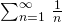# An interesting time complexity question

• Difficulty Level : Easy
• Last Updated : 30 Oct, 2015

What is the time complexity of following function fun()?

Attention reader! Don’t stop learning now. Get hold of all the important DSA concepts with the DSA Self Paced Course at a student-friendly price and become industry ready.  To complete your preparation from learning a language to DS Algo and many more,  please refer Complete Interview Preparation Course.

In case you wish to attend live classes with experts, please refer DSA Live Classes for Working Professionals and Competitive Programming Live for Students.

 `int` `fun(``int` `n)``{    ``    ``for` `(``int` `i = 1; i <= n; i++)``    ``{``        ``for` `(``int` `j = 1; j < n; j += i)``        ``{``            ``// Some O(1) task``        ``}``    ``}    ``}`

For i = 1, the inner loop is executed n times.
For i = 2, the inner loop is executed approximately n/2 times.
For i = 3, the inner loop is executed approximately n/3 times.
For i = 4, the inner loop is executed approximately n/4 times.
…………………………………………………….
…………………………………………………….
For i = n, the inner loop is executed approximately n/n times.

So the total time complexity of the above algorithm is (n + n/2 + n/3 + … + n/n)

Which becomes n * (1/1 + 1/2 + 1/3 + … + 1/n)

The important thing about series (1/1 + 1/2 + 1/3 + … + 1/n) is, it is equal to Θ(Logn) (See this for reference). So the time complexity of the above code is Θ(nLogn).

As a side note, the sum of infinite harmonic series is counterintuitive as the series diverges. The value ofis ∞. This is unlike geometric series as geometric series with ratio less than 1 converges.